# Editing¶

Reference

Mode

Edit Mode and Weight Paint Mode

Weights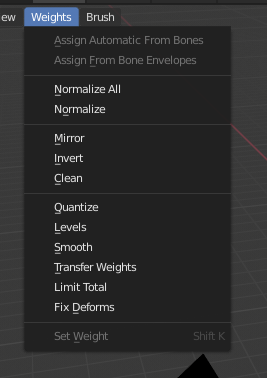Weight Paint Tools.

Blender provides a set of helper tools for Weight Painting.

The Subset Option

Some of the tools also provide a Subset filter to restrict their functionality to only specific vertex groups (in the Adjust Last Operation panel, displayed after the tool is called) with following options:

• Active Group

• Selected Pose Bones

• Deform Pose Bones

• All Groups

All tools also work with Vertex Selection Masking and Face Selection Masking. In these modes the tools operate only on selected vertices or faces.

## Assign from Bone Envelopes¶

Apply the envelope weight of the selected bone(s) to the selected vertex group.

## Assign Automatic from Bone¶

Apply from the selected bone(s) to the vertex group the same “auto-weighting” methods as available in the Parent armature menu.

## Normalize All¶

For each vertex, this tool makes sure that the sum of the weights across all vertex groups is equal to 1. This tool normalizes all of the vertex groups, except for locked groups, which keep their weight values untouched.

Lock Active

Keep the values of the active group while normalizing all the others.

## Normalize¶

This tool only works on the active vertex group. All vertices keep their relative weights, but the entire set of weights is scaled up such that the highest weight value is 1.0.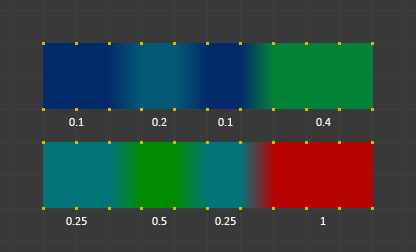Normalize example.

## Mirror¶

The Mirror Vertex Group tool mirrors the weights from one side of a perfectly symmetrical mesh to the opposite side. Only mirroring along local X axis is supported. Those vertices that have no corresponding vertex on the other side will not be affected. But note, the weights are not transferred to the corresponding opposite bone weight group.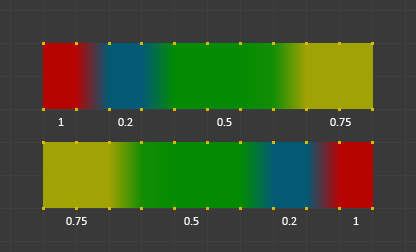Mirror example.

Mirror Weights

With this option checked, every selected vertex receives the weight information of its symmetrical counterpart. If both vertices are selected, it will be a weight information exchange; if only one is selected, information from the unselected will overwrite the selected one. Information on weight is passed for the active group only, unless All Groups is checked, in which case it is passed for all groups.

Flip Group Names

Works with selected vertices that belong to vertex groups with “symmetrical names” (with components like “L”, “R”, “right”, “left”). All selected vertices that belong to the active group, or to the symmetrical of the active group, will have their assignation to that group replaced by an assignation to the symmetrical one; however, its weight will be preserved. If All Groups is checked, all assignations to these kind of groups will be replaced by the symmetrical counterpart, also keeping the old weights.

All Groups

Operate on all vertex groups, instead of the active one.

Topology Mirror

Mirror for meshes which are not fully symmetric (approximate mirror). See here for more information.

Tip

Mirror to Opposite Bone

If you want to create a mirrored weight group for the opposite bone (of a symmetric character), then you can do this:

1. Delete the target vertex group (where the mirrored weights will be placed).

2. Create a copy of the source bone vertex group (the group containing the weights which you want to copy).

3. Rename the new vertex group to the name of the target vertex group (the group you deleted above).

4. Select the target vertex group and call the Mirror tool (use only the Mirror weights option and optionally Topology Mirror if your mesh is not symmetric).

## Invert¶

Replaces each Weight of the selected weight group by × -1.0 weight.

Examples:

• Original 1.0 converts to 0.0

• Original 0.5 remains 0.5

• Original 0.0 converts to 1.0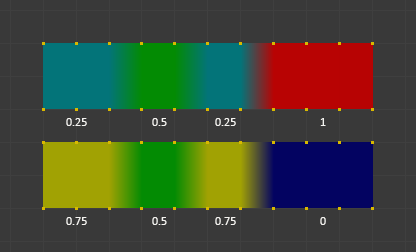Invert.

Subset

Restrict the tool to a subset. See above The Subset Option about how subsets are defined.

Add vertices that have no weight before inverting (these weights will all be set to 1.0).

Remove Weights

Remove vertices from the vertex group if they are 0.0 after inverting.

Note

Locked vertex groups are not affected.

## Clean¶

Clean Vertex Group Weights unassigns vertices from Vertex Groups whose weights are below the Limit. Removes weights below a given threshold. This tool is useful for clearing your weight groups of very low (or zero) weights.

In the example shown, a cutoff value of 0.2 is used (see operator options below) so all blue parts are cleaned out.

Note, the images use the Show Zero weights Active option so that unreferenced Weights are shown in Black.Clean example.

Subset

Restrict the tool to a subset. See above The Subset Option for how subsets are defined.

Limit

This is the minimum weight value that will be kept in the group. Weights below this value will be removed from the group.

Keep Single

Ensure that the Clean tool will not create completely unreferenced vertices (vertices which are not assigned to any vertex group), so each vertex will keep at least one weight, even if it is below the limit value!

## Quantize¶

This operator uses a process known as Quantization which takes the input weights and clamps each weight to a number of steps between (0 - 1), so there is no longer a smooth gradient between values.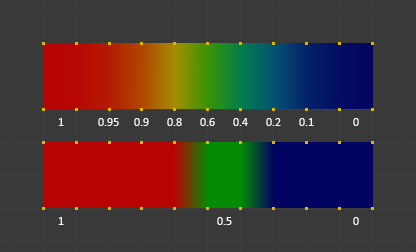Quantize example (Steps = 2).

Steps

The number of steps between 0 and 1 to quantize the weights into. For example 5 would allow the following weights [0.0, 0.2, 0.4, 0.6, 0.8, 1.0].

## Levels¶

Adds an offset and a scale to all weights of the selected weight groups. with this tool you can raise or lower the overall “heat” of the weight group.

Note

No weight will ever be set to values above 1.0 or below 0.0 regardless of the settings.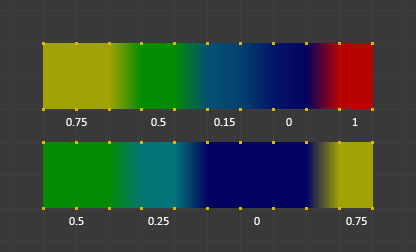Levels example.

Subset

Restrict the tool to a subset. See above The Subset Option for how subsets are defined.

Offset

A value from the range (-1.0 - 1.0) to be added to all weights in the vertex group.

Gain

All weights in the Subset are multiplied with the gain.

Note

Whichever Gain and Offset you choose, in all cases the final value of each weight will be clamped to the range (0.0 - 1.0). So you will never get negative weights or overheated areas (weight > 1.0) with this tool.

## Smooth¶

Tip

The Smooth tool only works when “Vertex selection masking for painting” is enabled. Otherwise the tool button is grayed out.

Blends the weights of selected vertices with adjacent unselected vertices. This tool only works in vertex select mode.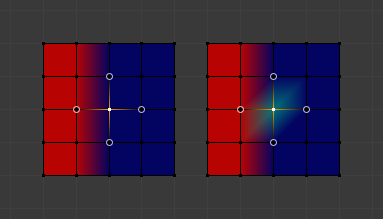To understand what the tool really does, let us take a look at a simple example. The selected vertex is connected to four adjacent vertices (marked with a gray circle in the image). All adjacent vertices are unselected. Now the tool calculates the average weight of all connected and unselected vertices. In the example this is:

$$(1 + 0 + 0 + 0) / 4 = 0.25$$

This value is multiplied by the factor given in the Operator options (see below).

• If the factor is 0.0 then actually nothing happens at all and the vertex just keeps its value.

• If the factor is 1.0 then the calculated average weight is taken (0.25 here).

• Dragging the factor from 0 to 1 gradually changes from the old value to the calculated average.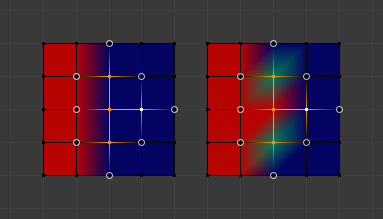Now let us see what happens when we select all but one of the neighbors of the selected vertex as well. Again all connected and unselected vertices are marked with a gray circle. When we call the Smooth tool now and set the Factor to 1.0, then we see different results for each of the selected vertices:

• The top-most and bottom-most selected vertices:

are surrounded by three unselected vertices, with an average weight of $$(1 + 0 + 0) / 3 = 0.333$$ So their color has changed to light green.

• The middle vertex:

is connected to one unselected vertex with weight = 1. So the average weight is 1.0 in this case, thus the selected vertex color has changed to red.

• The right vertex:

is surrounded by three unselected vertices with average weight = $$(0 + 0 + 0) / 3 = 0.0$$ So the average weight is 0, thus the selected vertex color has not changed at all (it was already blue before Smooth was applied).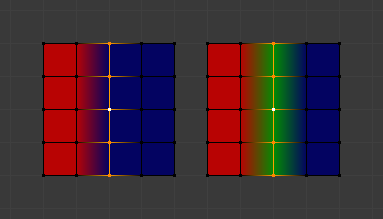Finally let us look at a practical example. The middle edge loop has been selected and it will be used for blending the left side to the right side of the area.

• All selected vertices have two unselected adjacent vertices.

• The average weight of the unselected vertices is $$(1 + 0) / 2 = 0.5$$

• Thus when the Factor is set to 1.0 then the edge loop turns to green and finally does blend the cold side (right) to the hot side (left).

Factor

The effective amount of blending. When Factor is set to 0.0 then the Smooth tool does not do anything. For Factor > 0 the weights of the affected vertices gradually shift from their original value towards the average weight of all connected and unselected vertices (see examples above).

Iterations

Number of times to repeat the smoothing operation.

Expand/Contract

Positive values expand the selection to neighboring vertices while contract limits to the selection.

Source

The vertices to mix with.

All

Smoothing will smooth both selected and deselected vertices.

Only Selected

Smoothing will only smooth with selected vertices.

Only Deselected

Smoothing will only smooth with deselected vertices.

## Transfer Weights¶

Copy weights from other objects to the vertex groups of the active object.

By default this tool copies only the active (selected) vertex group of the source object to the active vertex group of target object or creates a new one if the group does not exist. However, you can change the tool’s behavior in the Adjust Last Operation panel.

For example, to transfer all existing vertex groups from the source objects to the target, change the Source Layers Selection option to By Name.

Note

This tool uses the generic “data transfer”, but transfers from all selected objects to active one. Please refer to the Data Transfer docs for options details and explanations.

### Prepare the Copy¶

You first select all source objects, and finally the target object (the target object must be the active object).

It is important that the source objects and the target object are at the same location. If they are placed side-by-side, then the weight transfer will not work. (See the Vertex Mapping option.) You can place the objects on different layers, but you have to ensure that all objects are visible when you call the tool.

Now ensure that the target object is in Weight Paint Mode. Open the Toolbar and call the Transfer Weights tool in the Weight Tools panel.

### Adjust Last Operation Panel Confusion¶

You may notice that the Adjust Last Operation panel stays available after the weight transfer is done. The panel only disappears when you call another Operator that has its own Adjust Last Operation panel. This can lead to confusion when you use Transfer weights repeatedly after you changed your vertex groups. If you then use the still-visible Adjust Last Operation panel, then Blender will reset your work to its state right before you initially called the Transfer Weights tool.

So when you want to call the Transfer Weights tool again after you made some changes to your vertex groups, then always use the Transfer Weights button, even if the Adjust Last Operation panel is still available. Unless you really want to reset your changes to the initial call of the tool.

## Limit Total¶

Reduce the number of weight groups per vertex to the specified Limit. The tool removes lowest weights first until the limit is reached.

Hint

The tool can only work reasonably when more than one weight group is selected.

Subset

Restrict the tool to a subset. See above The Subset Option for how subsets are defined.

Limit

Maximum number of weights allowed on each vertex.

## Fix Deforms¶

The Fix deforms tool is used to modify an object’s nonzero weights so its deformed vertices are at a new defined distance. This is helpful to fix deformations because when complex models are deformed to their extreme poses, they are often visibly bumpy, jagged, or otherwise incorrectly deformed. Using this tool, you can smooth over the deformation.

To use the tool, select the vertices that you would like to move, either in Edit Mode or by using the vertex selection/mask. The operator can now be used and altered with these options:

Distance

The distance to move to.

Strength

The distance moved can be changed by this factor.

Accuracy

Changes the amount weights are altered with each iteration: lower values are slower.

Note

Note that if it does not change, then there are no nonzero bone weights that are changed to make it closer to the intended distance.

## Locks¶

Reference

Mode

Edit Mode and Weight Paint Mode

Weights ‣ Locks

Shortcut

K

Vertex groups can be locked to prevent undesired edits to a particular vertex group.

Tip

Bones that belong to a locked vertex group are displayed in red the 3D Viewport.

Lock All

Locks all vertex groups.

Unlock All

Unlocks all vertex groups.

Lock Selected

Locks selected vertex groups.

Unlock Selected

Unlocks selected vertex groups.

Lock Unselected

Locks unselected vertex groups.

Unlock Unselected

Unlocks Unselected vertex groups.

Lock Only Selected

Lock selected and unlock selected vertex groups.

Lock Only Unselected

Unlock selected and lock unselected vertex groups.

Invert Locks

Inverts the locks on all vertex groups.